# Units - math word problems

#### Number of problems found: 3477

• Supplementary angles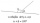One of the supplementary angles is larger by 33° than the second one. Calculate the angles size.
• Cube edgesIf the edge length of the cube increases by 50%, how does the volume of this cube increase?
• Equilateral triangleCalculate the side of an equilateral triangle, if its area is 892 mm2.
• Triangular pyramidWhat is the volume of a regular triangular pyramid with a side 3 cm long?
• Pump680 liters of water were pumped in 8 minutes. How many liters was spent in 56 minutes?On a straight stretch of road is marked 12 percent drop. What angle makes the direction of the road with the horizontal plane?
• Semicircle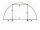To a semicircle with diameter 10 cm inscribe square. What is the length of square sides?
• Pentagon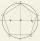Within a regular pentagon ABCDE point P is such that the triangle is equilateral ABP. How big is the angle BCP? Make a sketch.
• Estate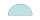Semicircle estate must be fence. The straight section has 26 meters long fence. How many meters of fence should buy?
• A bucketA bucket has 4 liters of water in it when it is 2/5 full. How much can it hold?
• Briefcase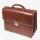The cost of producing briefcase is € 45. The manufacturer wants to sell it at a profit 30%. For how much will briefcase sell?
• QuartersHow many meters is a quarter of a kilometer? How many centimeters is a quarter of a meter, how many cents is a quarter of the euro?
• JagdeepJagdeep had a strip of paper measuring 1.8 m. He cut it into 4 equal strips. How long is each shorter strip of paper?
• BorrowingI borrow 25,000 to 6.9% p.a.. I pay 500 per month. How much will I pay and for how long?
• Vertices of RTShow that the points P1 (5,0), P2 (2,1) & P3 (4,7) are the vertices of a right triangle.
• GardenHow many steps of 76 cm circumvent square garden with area 1.8 ha?
• Each chair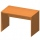Each chair costs twice less than two tables. One table costs 720 Czech crowns. How many crowns are the table and 4 chairs?
• The numberThe number of 1 cm cubes required to make 4 cm cube is?
• Triangle ABCTriangle ABC has side lengths m-1, m-2, m-3. What has to be m to be triangle a) rectangular b) acute-angled?
• Akpan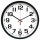Akpan spent 3/8 of his time in school during the week. What fraction of his time does he spend at home during the week?

Do you have an interesting mathematical word problem that you can't solve it? Submit a math problem, and we can try to solve it.

We will send a solution to your e-mail address. Solved examples are also published here. Please enter the e-mail correctly and check whether you don't have a full mailbox.

Please do not submit problems from current active competitions such as Mathematical Olympiad, correspondence seminars etc...